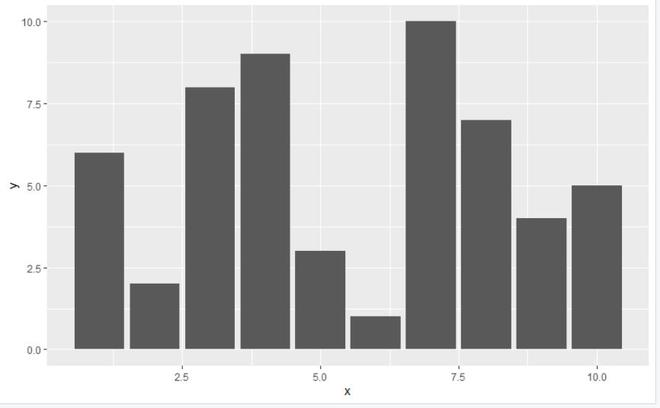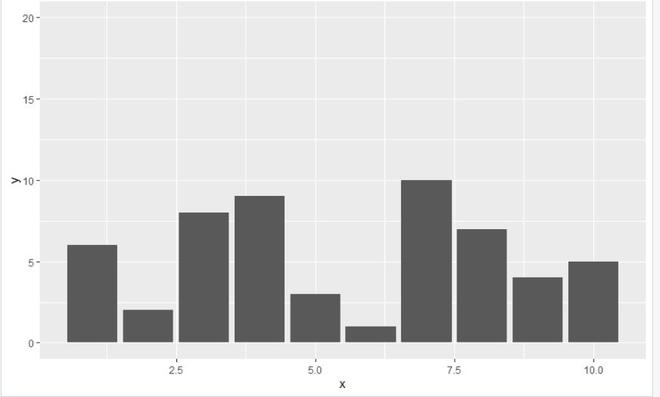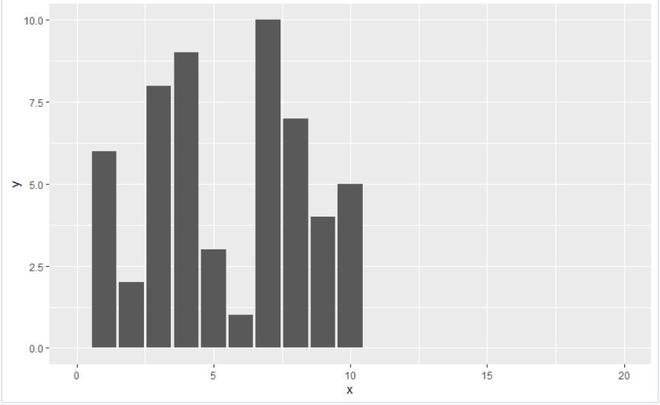# Set ggplot2 Axis Limit Only on One Side in R

• Last Updated : 08 Oct, 2021

In this article, we are going to set the ggplot2 plot axis limit only on one side of the plot in the R programming language.

## Using scale_x_continuous() or scale_y_continuous() function

scale_x_continuous()/scale_y_continuous() function: This function is for the default scales for continuous x or y aesthetics.

Syntax: scale_y_continuous(name = waiver(), breaks = waiver(), minor_breaks = waiver(), labels = waiver(), limits = NULL,expand = waiver(), oob = censor, na.value = NA_real_,trans = “identity”)

Parameters:

• name: The name of the scale. Used as axis or legend title.
• limits: A numeric vector of length two providing limits of the scale. Use NA to refer to the existing minimum or maximum.
• expand: A numeric vector of length two giving multiplicative and additive expansion constants.
• oob: Function that handles limits outside of the scale limits (out of bounds). The default replaces out-of-bounds values with NA.
• na.value: Missing values will be replaced with this value.
• trans: Either the name of a transformation object, or the object itself.
• …: Other arguments passed on to scale_(x|y)_continuous

## Change Only One Axis Limit Using scale_y_continuous:

In this example, we will be plotting a ggplot2 plot of 10 data points for setting up the axis limit of only one axis which will be of y-axis using scale_y_continuous() function in the R programming language.

Initial plot with given data points look as without any changes:

## R

 `library``(ggplot2)` `data < - ``data.frame``(x=``c``(4, 9, 5, 6, 10, 2, 3, 7, 8, 1),``                    ``y=``c``(9, 4, 3, 1, 5, 2, 8, 10, 7, 6))` `plot < - ``ggplot``(data, ``aes``(x, y)) +``geom_bar``(stat=``"identity"``)` `plot`

Output:## R

 `library``(ggplot2)` `data < - ``data.frame``(x=``c``(4, 9, 5, 6, 10, 2, 3, 7, 8, 1),``                    ``y=``c``(9, 4, 3, 1, 5, 2, 8, 10, 7, 6))` `plot < - ``ggplot``(data, ``aes``(x, y)) +``geom_bar``(stat=``"identity"``) +``scale_y_continuous``(limits=``c``(0, 20))` `plot`

Output:## Change Only One Axis Limit Using scale_x_continuous()

In this example, we will be plotting a ggplot2 plot of 10 data points for setting up the axis limit of only one axis which will be of x-axis using scale_x_continuous() function in the R programming language.

## R

 `library``(ggplot2)` `data < - ``data.frame``(x=``c``(4, 9, 5, 6, 10, 2, 3, 7, 8, 1),``                    ``y=``c``(9, 4, 3, 1, 5, 2, 8, 10, 7, 6))` `plot < - ``ggplot``(data, ``aes``(x, y)) +``geom_bar``(stat=``"identity"``) +``scale_x_continuous``(limits=``c``(0, 20))` `plot`

Output:My Personal Notes arrow_drop_up